# Heath-Brown: Rational Points and Analytic Number Theory

Analytic number theory is often quite useful in questions on rational points on varieties. For example, using the circle method, weak approximation gives formulas of the type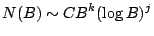where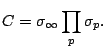What kind of asymptotic formulae can we expect when weak approximation fails?

Look at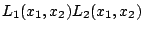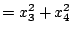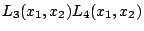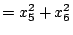where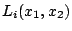are linear forms over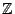. This is the intersection of two quadrics in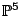. In this case, the Hasse principle may fail, and weak approximation may fail. We take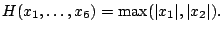We have the following theorem'': There is a modification of the Hardy-Littlewood formula in which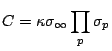where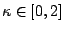,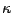vanishes precisely when the Hasse principle fails,is built from information at a finite number of bad' places, and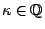is easily calculable. To do this, use a `descent'' process followed by variation of the circle method.

Theorem. [H-B, Moroz] Let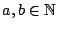be coprime, with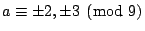. Then the surface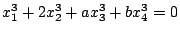has a nontrivial rational point.

To prove this, there are two ingredients. First, a result of Satgé: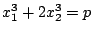has a rational point if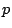is prime,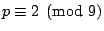(proved by Heegner point construction). Second,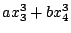takes infinitely many prime values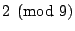.

In the other direction, analytic number theorists are often interested in rational points on varieties. We take the counting function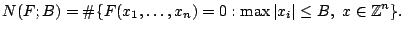For instance, take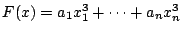; there exists an asymptotic formula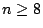(Vaughan). In the case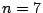, analytic methods establish a local-global principle, but not an asymptotic formula. To handle this case, we would want: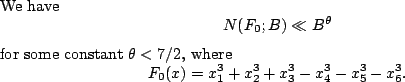This is known for any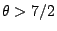, and conjectured to be true for any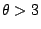, so it is reasonable to expect.

What about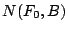for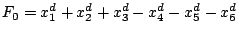? We can show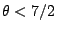for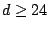. This variety has lines in trivial planes of the type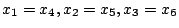, and no other lines if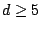; what other quadric or low degree curves can be found?

Proposition. [Green 1975] Any curve of genus 0 or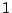in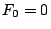has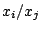constant for some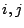, as soon as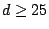.

(Here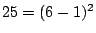.) This involves meromorphic functions and Nevanlinna theory.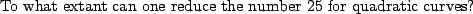Proposition. [Davenport 1963] Any cubic form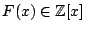in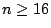variables has a nontrivial integer zero.

This applies to an arbitrary cubic form; there is a better result for smooth forms with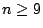. Define a matrix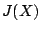with entries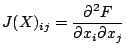and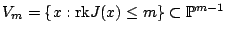. Assume that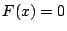has no nontrivial rational point; then any component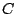of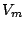which has a rational point has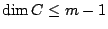.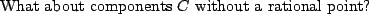Vinogradov's mean value theorem refers to the counting function of the variety defined by the equations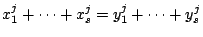for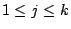,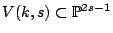, count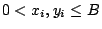. This is a cone with vertex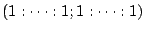.

One can easily show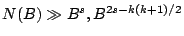for all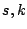; if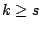then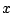is a permutation of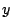,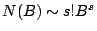. In fact,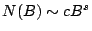for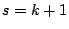(Vaughan, Wooley).

For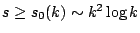, we have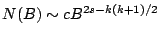.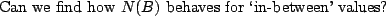Applications: Exponential sums, zero-free region for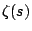and the error term in the prime number theorem. So this question has several far-reaching implications!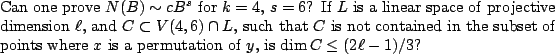Back to the main index for Rational and integral points on higher dimensional varieties.